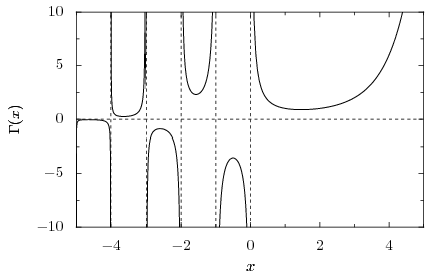Wednesday, January 9, 2013

Behavior of the Gamma Function

In the previous post, the idea of the Gamma function, or the extension of the factorial beyond the positive integers, was introduced. In addition, an expression for it, in the form of an infinite product, was discussed. But how does the function actually behave outside of the integers? First, we shall consider some basic facts:

We have already confirmed that the factorial function grows faster than the polynomial and exponential functions. In addition, it grows slower than the function xx, a fact which can be confirmed without difficulty in a similar manner.

Also of importance is the total determination of the function on the real numbers given only its value on the interval (0,1) (the real numbers from 0 to 1 not including 0 or 1). This is due to one of the two fundamental properties of the Gamma function: Γ(x + 1) = xΓ(x). Since any real number can be expressed as the sum of an integer and a number between zero and one, iterated applications of the above property will yield the desired value. For example,

Γ(3.5) = (2.5)Γ(2.5) = (2.5)(1.5)Γ(1.5) = (2.5)(1.5)(0.5)Γ(0.5), thus reducing the problem of calculating the value of the function over the real numbers to that of calculating it only between zero and one.

Of the values on (0,1), all can be approximated numerically to any degree of accuracy with the infinite product, but only one, Γ(1/2), is known in closed form. Its exact value is

Γ(1/2) = (π)1/2, a fact that was discovered when a previously known infinite product that equaled π was identified with the expression when 1/2 is substituted in for the definition of Γ(x). Thus, any real number with fractional part 1/2 can be expressed in closed form. The graph of the Gamma function on the real line is as shown below.It is clear that the Gamma function is undefined at each non-positive integer, as is consistent with the definition. In addition, there is no number x such that Γ(x) = 0. The graph alternates between positive and negative on the intervals between the negative integers because going from one interval to the next is equivalent to multiplying (or dividing) by a negative number in accordance with the above rule.

However, the Gamma function is not even limited to the real line. It is also defined for all complex values using the same infinite product as before. The product is defined at all complex numbers off the real line, i.e. there are no additional discontinuities beyond the non-positive integers. The behavior of the Gamma function over the entire complex plane is illustrated below.To graph such a function on the complex plane would normally require four axes, two for the input z, the real part and the imaginary part, and two for the output Γ(z). Since there are only two dimensions available when graphing on a plane, hue and lightness are used in addition to illustrate the behavior of the function. The two physical dimensions of the graph illustrate the position of the input on the complex plane in accordance with the numbered axes (horizontal: real axis, vertical: imaginary axis). The output is colored according to the scheme illustrated below:

The modulus of the complex number is indicated by its lightness; numbers closer to zero are indicated by darker colors, while complex numbers far from zero are whiter. Furthermore, the argument, or angle made with the positive horizontal axis, is indicated by hue. For example, a complex number with an argument near zero appears red, while one with an argument near π (180°) is blue. Returning to the complex "graph" of the Gamma Function, it is easy to see how the graph on the real line is a cross section of the full graph: along the horizontal real axis in the middle, the output is red (positive real number, argument 0) for positive input, and alternating between red and light blue (negative real number, argument π) for negative integers. Also, as one travels further negative, the outputs become very small (darker), except for the spike at each negative integer where the function goes to infinity (white dots). The points at which the Gamma function is undefined are called poles.

Over the rest of the complex plane, it can be seen that the function's value at any complex number is the conjugate (reflection over the real axis) of the value at its conjugate. In other words, the output at two points that are reflections over the real axis have the "opposite" colors indicated by the scheme above. In equation form,

All of these properties are illustrated on a copy of the complex graph above:
Due to its nature as an extension of the factorials, the Gamma function allows an analogous broadening of the field of probability theory. It also finds applications related to binomials and the evaluation of coefficients in a product. Finally, it is related to other functions involved in number theory, and emerges in computations in a variety of mathematical fields.

Sources: Gamma Function, Wikipedia, Mathematical Thought from Ancient to Modern Times (Vol. 2) by Morris Kline